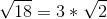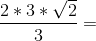## Example Questions

### Example Question #12 : Squaring / Square Roots / Radicals

Which real number satisfies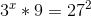?Explanation:

Simplify the base of 9 and 27 in order to have a common base.

(3x)(9)=272

= (3x)(32)=(33)2

=(3x+2)=36

Therefore:

x+2=6

x=4

### Example Question #13 : Squaring / Square Roots / Radicals

Which of the following is a factor of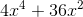?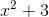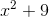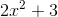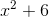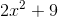Explanation:

The terms ofhave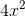as their greatest common factor, so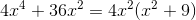is a prime polynomial.

Of the five choices, onlyis a factor.

### Example Question #14 : Squaring / Square Roots / Radicals

Simplify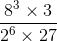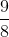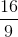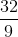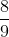Explanation:

The easiest way to approach this problem is to break everything into exponents.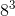is equal to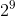and 27 is equal to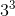. Therefore, the expression can be broken down into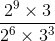. When you cancel out all the terms, you get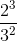, which equals.

### Example Question #8 : Squaring / Square Roots / Radicals

Which of the following expression is equal to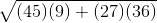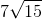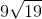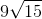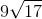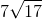Explanation:When simplifying a square root, consider the factors of each of its component parts: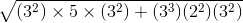Combine like terms: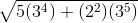Remove the common factor,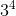: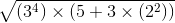Pull the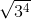outside of the equation as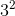: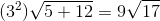### Example Question #9 : Squaring / Square Roots / Radicals

Which of the following is equal to the following expression?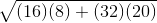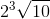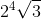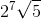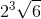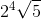Explanation:First, break down the components of the square root: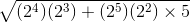Combine like terms. Remember, when multiplying exponents, add them together: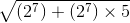Factor out the common factor of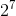: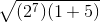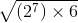Factor the: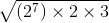Combine the factoredwith the: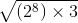Now, you can pull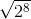out from underneath the square root sign as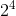:### Example Question #10 : Squaring / Square Roots / Radicals

Which of the following expressions is equal to the following expression?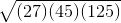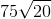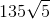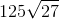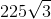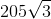Explanation:First, break down the component parts of the square root: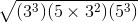Combine like terms in a way that will let you pull some of them out from underneath the square root symbol: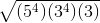Pull out the terms with even exponents and simplify: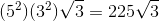### Example Question #15 : Squaring / Square Roots / Radicals

What is,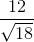?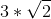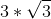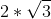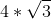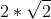Explanation:

To find an equivalency we must rationalize the denominator.

To rationalize the denominator multiply the numerator and denominator by the denominator.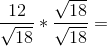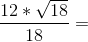Factor out 6,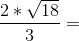Extract perfect square 9 from the square root of 18.